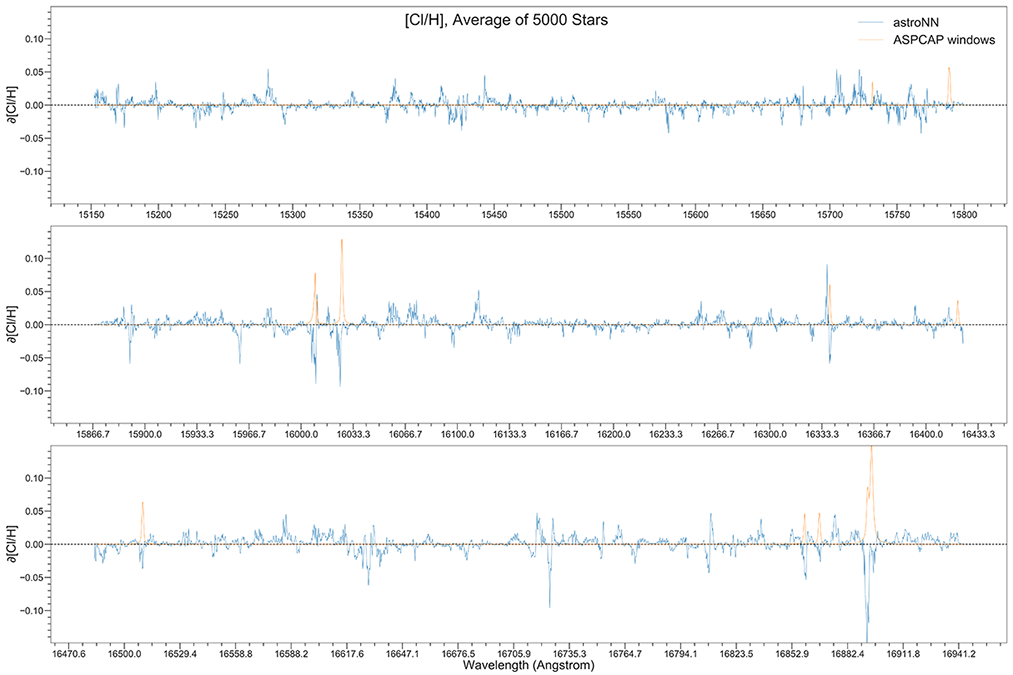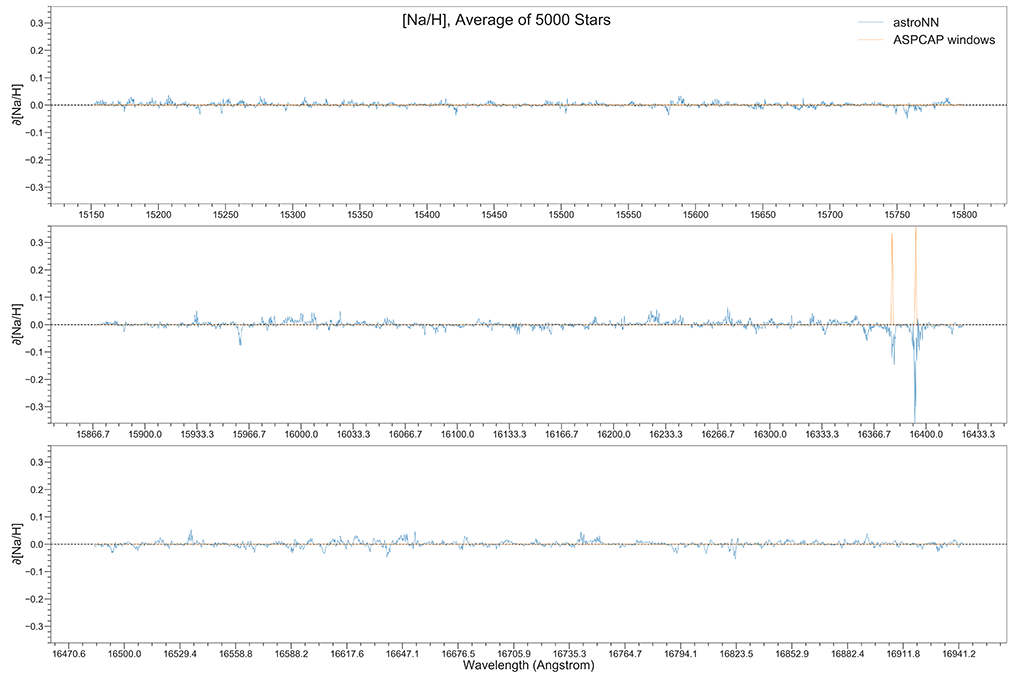# APOGEE Spectra with Convolutional Neural Net - astroNN.models.ApogeeCNN¶

Warning

Please refer to Bayesian Neural Network for the most updated result: http://astronn.readthedocs.io/en/latest/neuralnets/apogee_bcnn.html

class astroNN.models.ApogeeCNN.ApogeeCNN(lr=0.005)

Class for Convolutional Neural Network for stellar spectra analysis

History: 2017-Dec-21 - Written - Henry Leung (University of Toronto)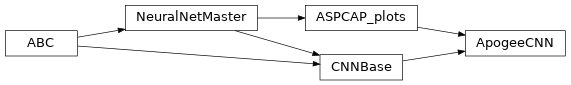Although in theory you can feed any 1D data to astroNN neural networks. This tutorial will only focus on spectra analysis.

from astroNN.models import ApogeeCNN

# Load the train data from dataset first, x_train is spectra and y_train will be ASPCAP labels

# And then create an instance of Convolutional Neural Network class
cnn_net = ApogeeCNN()

# You don't have to specify the task because its 'regression' by default. But if you are doing classification. you can set task='classification'

# Set max_epochs to 10 for a quick result. You should train more epochs normally
cnn_net.max_epochs = 10
cnn_net.train(x_train, y_train)


Here is a list of parameter you can set but you can also not set them to use default

ApogeeCNN.batch_size = 64
ApogeeCNN.initializer = 'he_normal'
ApogeeCNN.activation = 'relu'
ApogeeCNN.num_filters = [2, 4]
ApogeeCNN.filter_len = 8
ApogeeCNN.pool_length = 4
ApogeeCNN.num_hidden = [196, 96]
ApogeeCNN.max_epochs = 250
ApogeeCNN.lr = 0.005
ApogeeCNN.reduce_lr_epsilon = 0.00005
ApogeeCNN.reduce_lr_min = 0.0000000001
ApogeeCNN.reduce_lr_patience = 10
ApogeeCNN.target = 'all'
ApogeeCNN.l2 = 1e-7
ApogeeCNN.input_norm_mode = 1
ApogeeCNN.labels_norm_mode = 2


Note

You can disable astroNN data normalization via ApogeeCNN.input_norm_mode=0 as well as ApogeeCNN.labels_norm_mode = 0 and do normalization yourself. But make sure you don’t normalize labels with MAGIC_NUMBER (missing labels).

After the training, you can use cnn_net in this case and call test method to test the neural network on test data. Or you can load the folder by

from astroNN.models import load_folder

# Load the test data from dataset, x_test is spectra and y_test will be ASPCAP labels

pred = cnn_net.test(x_test)  # pred contains denormalized result aka. ASPCAP labels prediction in this case


Since astroNN.models.ApogeeCNN does not have uncertainty analysis feature. You can plot aspcap label residue by supplying zeros arrays as error value. If you want model uncertainty/ risk estimation and propagated error, please use astroNN.models.ApogeeBCNN.

import numpy as np
cnn_net.aspcap_residue_plot(pred, y_test, np.zeros(y_test.shape))


You can calculate jacobian which represents the output derivative to the input and see where those output is sensitive to in inputs.

# Calculate jacobian first
jacobian_array = cnn_net.jacobian(x_test, mean_output=True)

# Plot the graphs
cnn_net.jacobian_aspcap(jacobian=jacobian_array, dr=14)


Note

You can access to Keras model method like model.predict via (in the above tutorial) cnn_net.keras_model (Example: cnn_net.keras_model.predict())

## Example Plots using aspcap_residue_plot¶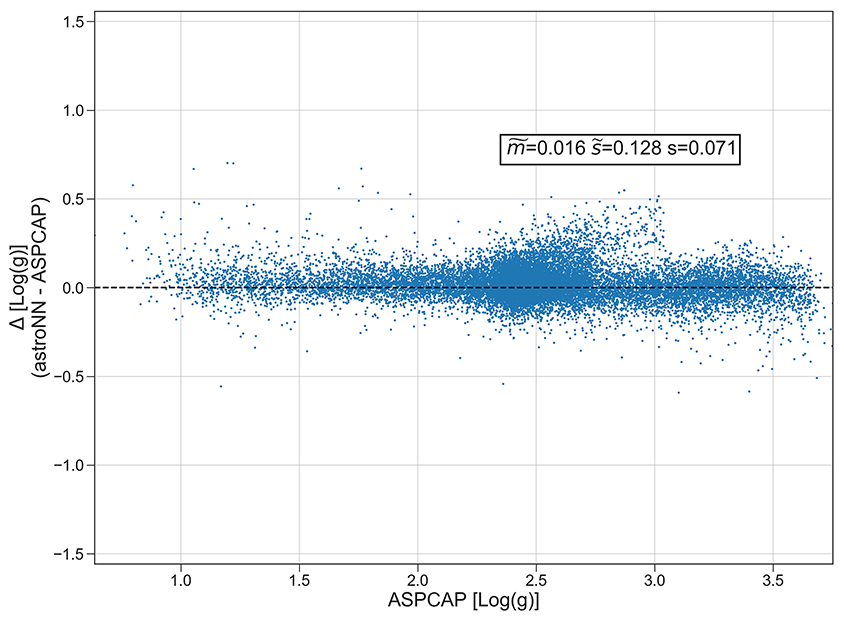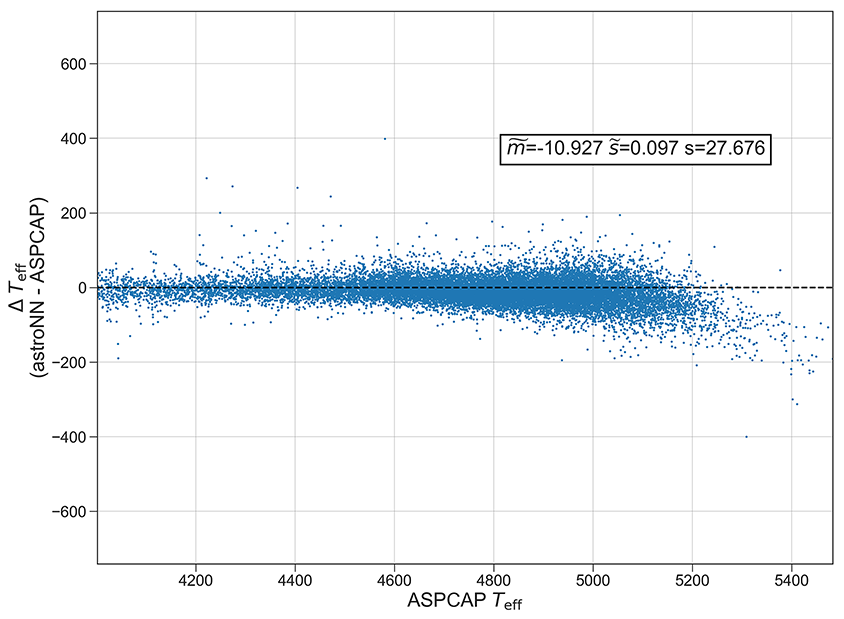## ASPCAP labels prediction using CNN vs The Cannon 2¶

Warning

Please refer to Bayesian Neural Network for the most updated result: http://astronn.readthedocs.io/en/latest/neuralnets/apogee_bcnn.html## Example Plots using jacobian¶